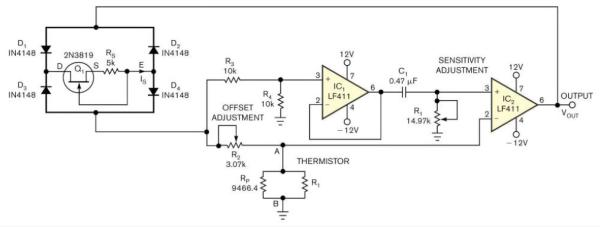# Temperature-to-period circuit provides linearization of thermistor response

Designers often use thermistors rather than other temperature sensors because thermistors offer high sensitivity, compactness, low cost, and small time constants. But most thermistors’ resistance-versus-temperature characteristics are highly nonlinear and need correction for applications that require a linear response. Using a thermistor as a sensor, the simple circuit in Figure 1 provides a time period varying linearly with temperature with a nonlinearity error of less than 0.1K over a range as high as 30K. You can use a frequency counter to convert the period into a digital output. An approximation derived from Bosson’s Law for thermistor resistance, RT, as a function of temperature, θ, comprises RT=AB–θ (see sidebar “Exploring Bosson’s Law and its equation”). This relationship closely represents an actual thermistor’s behavior over a narrow temperature range.Figure 1

This simple circuit linearizes a thermistor’s response and produces an output period that’s proportional to temperature.

You can connect a parallel resistance, RP, of appropriate value across the thermistor and obtain an effective resistance that tracks fairly close to AB–θ30K. In Figure 1, the network connected between terminals A and B provides an effective resistance of RABAB–θ. JFET Q1 and resistance RS form a current regulator that supplies a constant current sink, IS, between terminals D and E.

Through buffer-amplifier IC1, the voltage across R4 excites the RC circuit comprising R1 and C1 in series, producing an exponentially decaying voltage across R1 when R2 is greater than RAB. At the instant when the decaying voltage across R1 falls below the voltage across thermistor RT, the output of comparator IC2changes its state. The circuit oscillates, producing the voltage waveforms in Figure 2 at IC2‘s output. The period of oscillation, T, is T=2R1C1ln(R2/RAB)2R1C1[ln(R2/A)+θlnB]. This equation indicates that T varies linearly with thermistor temperature θ.